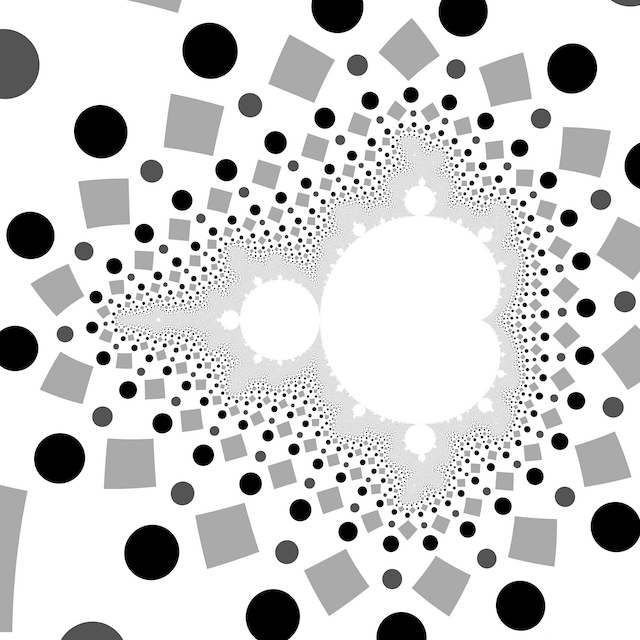# # Exterior CoordinatesEscape radius around $$R = 25^2$$ makes the exterior grid cells roughly square. The imaginary part of $$e$$ is linearized to avoid shape distortion. $\Re e = \frac{\arg z}{2 \pi} \mod 1$ $\Im e = 2 - \frac{\log \left|z\right|}{\log R}$

The exterior coordinate $$e$$ can be used to look up pixel colours from an image texture, but care must be taken to avoid glitches at the cell boundaries if automatic derivatives are used for mipmapped anti-aliasing. The calculation of the derivatives must be modified to account for the wrapped space.

Dancing Angels (2012) has an example of animating image texture during a zoom video.

## # 1 C99 Code

#include <complex.h>
#include <math.h>

const double pi = 3.141592653589793;

double _Complex m_exterior_coordinates(int N, double R, double _Complex c)
{
double _Complex z = 0;
for (int n = 0; n < N; ++n)
{
if (cabs(z) > R)
return fmod(1 + carg(z) / (2 * pi), 1)
+ I * (2 - log(cabs(z)) / log(R));
z = z * z + c;
}
return 0;
}